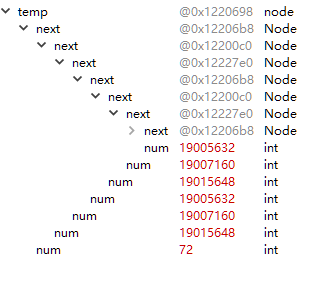C 指针问题求助大神，程序为链表的选择排序，我的方法为将最小的节点放到头节点之后

`````` #include<stdio.h>
#include<stdlib.h>
#include<stdlib.h>
#include<time.h>

typedef struct Node
{
int num;
struct Node* next;

}node;

int main()
{
srand(time(0));
node *temp=(node*) malloc(sizeof(node));
for(int i=0;i<2;i++)
{
item=(node*) malloc(sizeof(node));
item->num=rand()%100;
item->next=NULL;
temp->next=item;
temp=item;
}
free(item);
free(temp);
//    node* item;
//    while(item!=NULL)
//    {
//        printf("%d,",item->num);
//        item=item->next;
//    }
//    printf("\n");
node* min=(node*) malloc(sizeof(node));
//    node* temp=(node*) malloc(sizeof(node));
while(item->next)
{
free(temp);
for(temp=min;(temp->next)!=NULL;)  //调试过程中运行到这一步temp变的很奇怪是什么原因？调试截图在下面
{
if(temp->next->num<min->num)
{
min=temp;
}
temp=temp->next;
}
temp=min->next;
min->next->next=temp->next;
item=item->next;
}
while(item!=NULL)
{
printf("%d,",item->num);
item=item->next;
}
}
``````2个回答

temp=item ，已经释放了item就不要释放temp因为它们指向的是一块内存地址